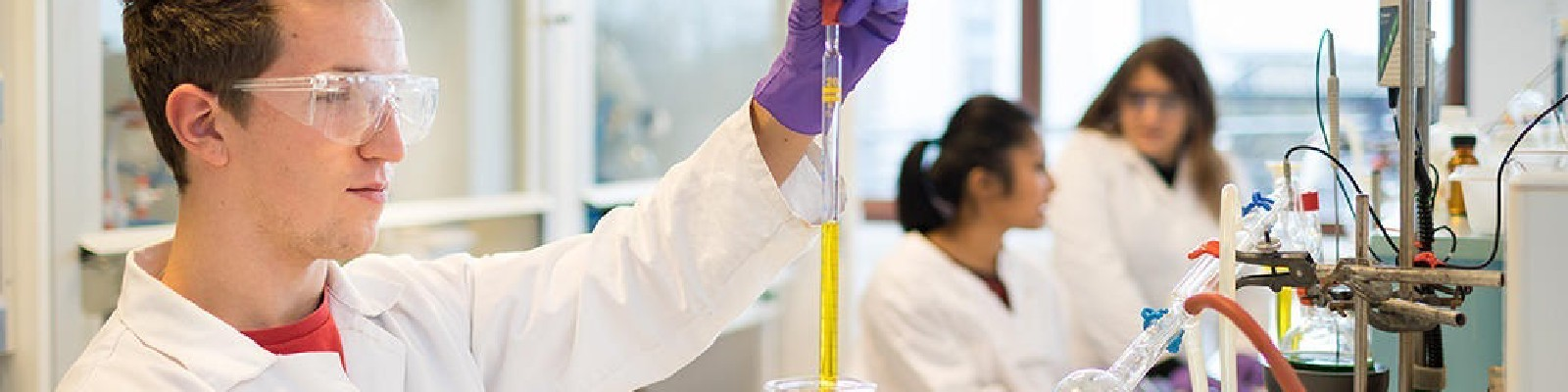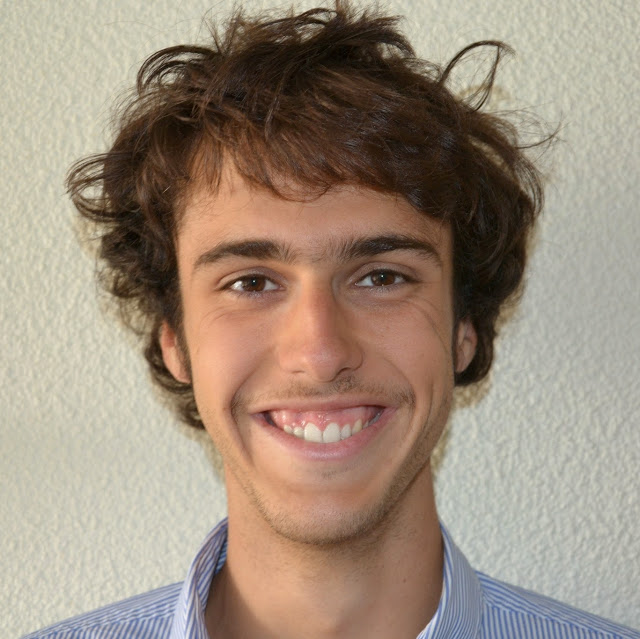Student in bio-chemistry courses © Arthur Pequin

# Overview

The Health and Science is established since 2107 and proposes to the students of second year medical studies, pharmacology and odontology an early formation to research.

# Course

• Physical problem example: two weights bound with a spring. System of two bounded ordinary differntial equations (EDO). Solution with no linear algebra general theory.

• Linear algebra discovering: vectors, matrices, basis operations: sum, product, trace, determinant, inverse. Notions of free family. Diagonalisation.

• Two applied examples:

• Square inverse problem (as much equations as variables): solution with matrix inversion.
• Solve a system of coupled linear EDO thanks to diagonalisation.

# Practical work on Python

• General formalism over Python for vectors and matrices.
• How can we do the loops ?
• Square linear problem resolution.
• Eigen-space decomposition.
• Numerical solution of the problem: two weights bound with a spring.